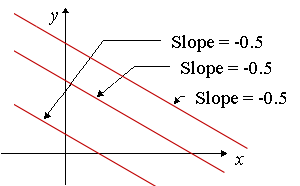Search IntMath
Close

450+ Math Lessons written by Math Professors and Teachers

5 Million+ Students Helped Each Year

1200+ Articles Written by Math Educators and Enthusiasts

Simplifying and Teaching Math for Over 23 Years

# Parallel LinesParallel lines have the same slope.

Mathematically, we say if one line has slope m1 and another line has slope m2 then the lines are parallel if

m1 = m2

## Interactive Graph

You can explore the concept of parallel lines in the following JSXGraph (it's not a fixed image).

Drag either point A or point B to explore slopes of parallel lines.

You can move the graph up-down, left-right if you hold down the "Shift" key and then drag the graph.

If you get lost, you can always refresh the page.

## Problem SolverThis tool combines the power of mathematical computation engine that excels at solving mathematical formulas with the power of GPT large language models to parse and generate natural language. This creates math problem solver thats more accurate than ChatGPT, more flexible than a calculator, and faster answers than a human tutor. Learn More.